# Motivations and goals

In this week’s lesson, we return to `ggplot()` to further expand our data visualization toolkit. The material we cover this week will help you make clearer (and more beautiful!) plots for your presentaitons and papers.

# Set-up

Start by downloading the the tidyverse package if you haven’t done so yet. You can install the package by typing `install.packages("tidyverse")` into the console of RStudio and then hitting enter.

Then load the tidyverse as follows:

``library(tidyverse)``

Like last week, we’re going to use the data from the `fivethirtyeight()` package. So, install if needed (`install.packages("fivethirtyeight")`) and then load that package as well.

``library(fivethirtyeight)``

# Refresher: ggplot basics

We’re going to start by looking at the dataset `comma_survey`, which contains data from a survey of Americans on their opinions of the Oxford comma and other grammar-related questions. You can look at the dataset using `View()`. You can see a list of the variable names using `names()`.

``````View(comma_survey)
names(comma_survey)``````

Let’s make a simple bar chart showing how much respondents care about the Oxford comma:

``````# We start by just telling gpplot() which dataset and variables we're using:
ggplot(comma_survey, aes(x=care_oxford_comma)) +

# Then, we say what type of geom(s) we want to use:
geom_bar() +

# Then, we add a title:
ggtitle("How much do people care about Oxford commas?") ``````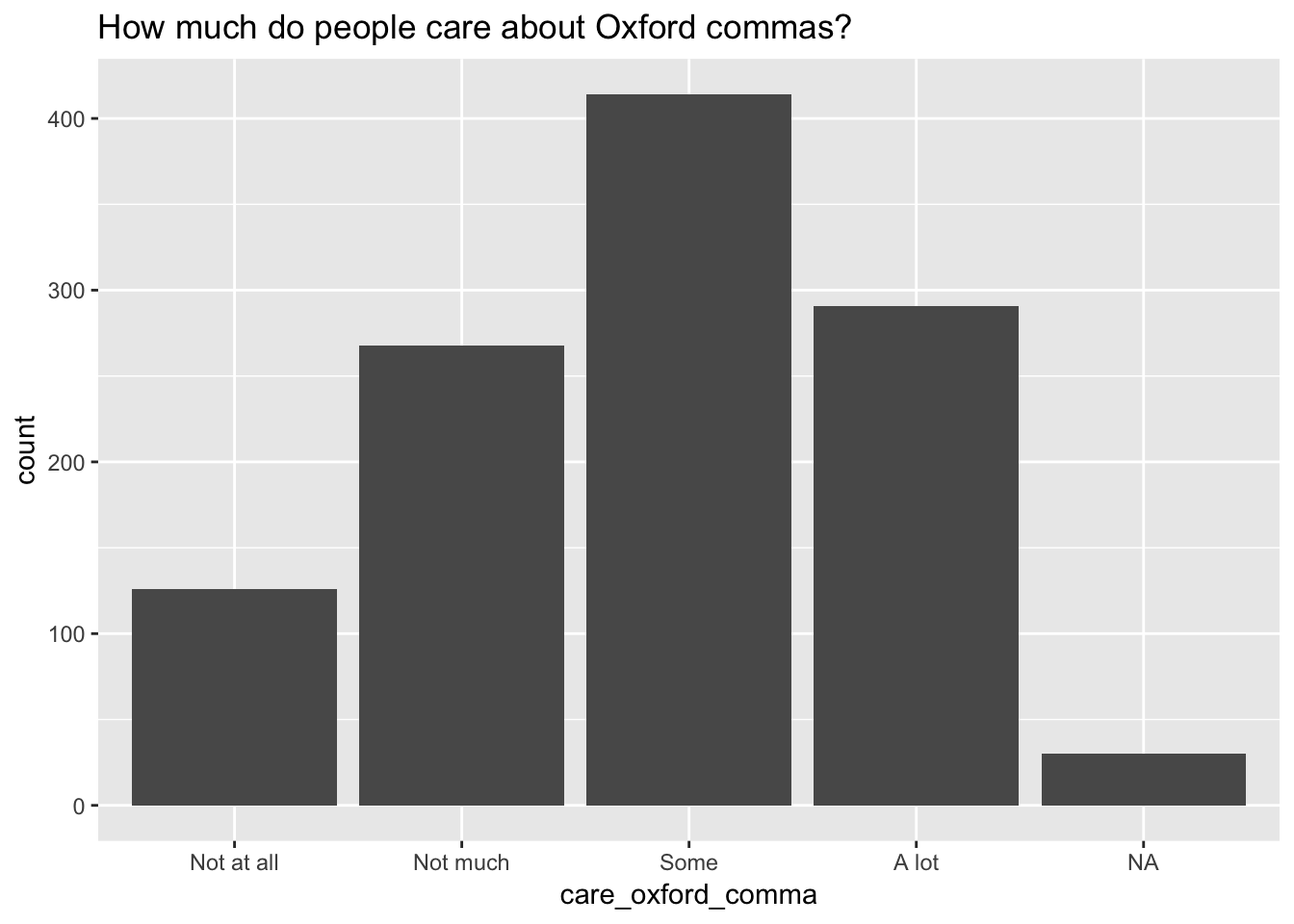We can clean this up a little bit by removing the missing values, changing the y-axis to a percentage instead of a count, and getting rid of the unnecessary x-axis label:

``````comma_survey %>%
filter(!is.na(care_oxford_comma)) %>%
ggplot(aes(x=care_oxford_comma)) +

# This line of code puts the percentage on the y-axis:
geom_bar(aes(y = (..count..)/sum(..count..)*100)) +
ggtitle("How much do people care about Oxford commas?") + xlab("") + ylab("Percent")``````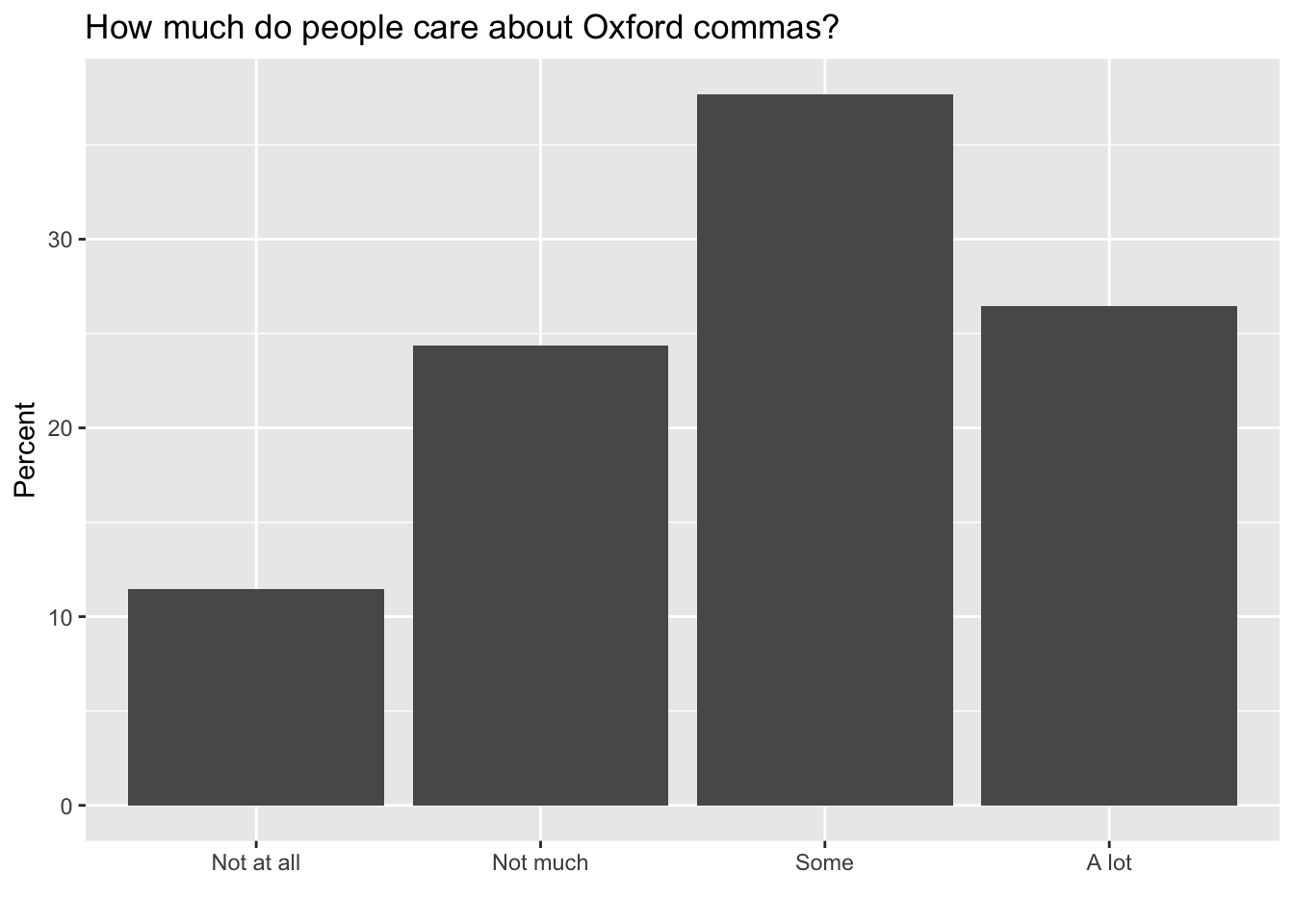Other geoms we have seen so far include: `geom_histogram()`, `geom_line()` and `geom_point()`.

# Creating the same plot for different subgroups of data using facet_wrap()

We often want to visualize our data for different subgroups of the population. `ggplot()` with `facet_wrap()` allows us to create different “facets” of our plot for different subgroups of the population. For example, let’s say we’re interested in comparing how much people care about Oxford commas, based on whether or not they actually use them. We can do this as follows:

``````comma_survey %>%
filter(!is.na(care_oxford_comma)) %>%
ggplot(aes(x=care_oxford_comma)) +

# This line of code puts the percentage on the y-axis:
geom_bar(aes(y = (..count..)/sum(..count..)*100)) +

# This line of code adds "facets" based on which sentence people thought was grammatically correct:
facet_wrap(~more_grammar_correct) +
ggtitle("How much do people care about Oxford commas?") + xlab("") + ylab("Percent")``````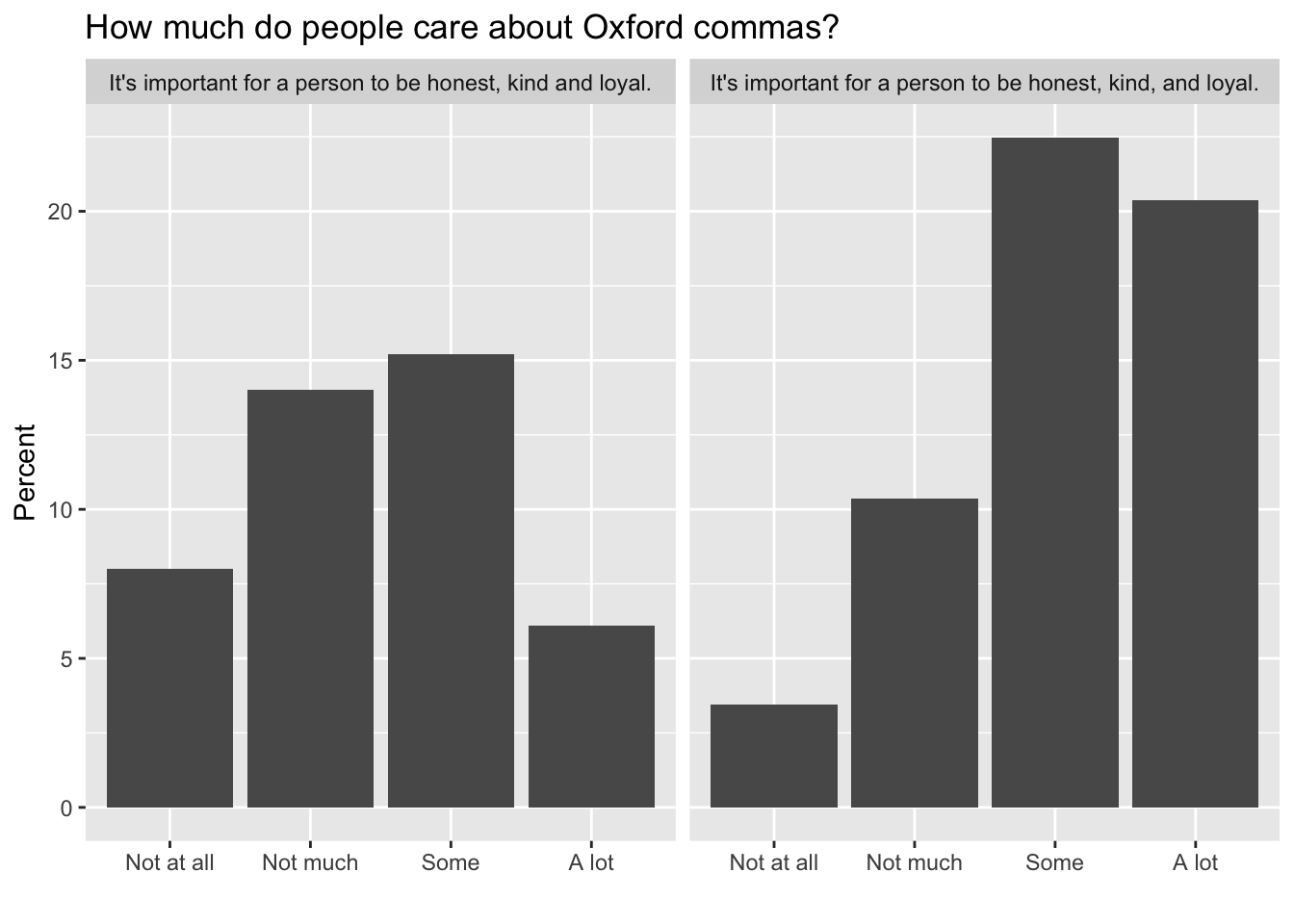It looks like people who use Oxford commas have much stronger feelings about them than people who don’t use them!

We may be interested in whether Oxford comma use varies with age. We can look at this using a scatter plot:

``````comma_survey %>%

# First, get rid of observations that are missing either of the two variables we're interested in:
filter(!is.na(care_oxford_comma) & !is.na(age)) %>%

# Next, create a numeric variable for whether the respondent used the Oxford comma
mutate(oc_use = ifelse(more_grammar_correct=="It's important for a person to be honest, kind and loyal.", 0, 1)) %>%

# Next, calculate the % of respondents who used the Oxford comma within each age group
group_by(age) %>% summarize (oc_use = mean(oc_use, na.rm=T)) %>%

# Finally, make the plot:
ggplot(aes(x=age, y=oc_use, group=1)) +
# note that we need to indicate "group=1" in order to draw a line here (because the x-variable is a factor, and ggplot usually does not allow us to draw lines when the x-variable is a factor unless we indicate that there is one group)

geom_point() + geom_line() +
ggtitle("How does Oxford comma use vary with age") + xlab("Age") + ylab("Oxford comma use")``````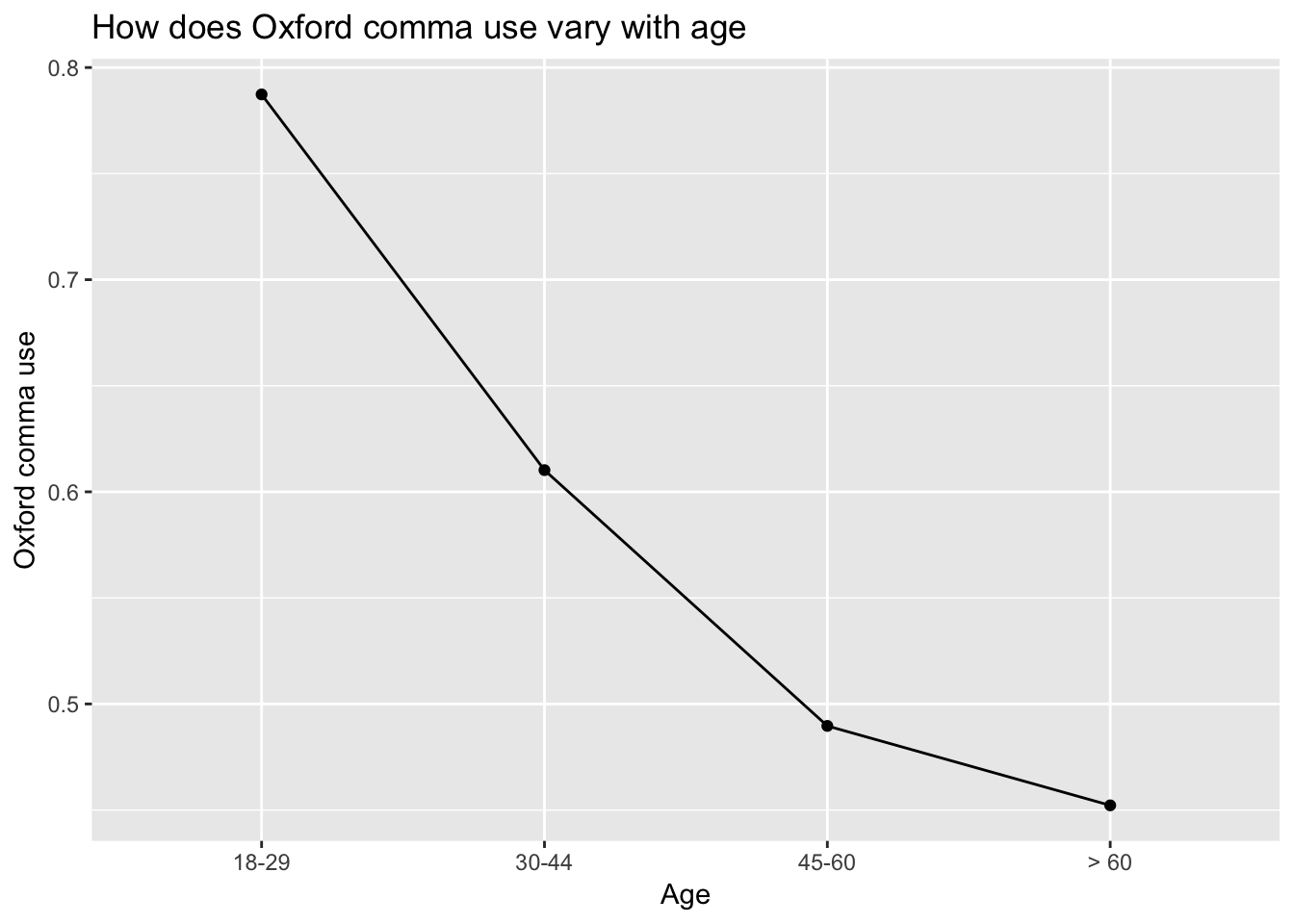Now, let’s look at this relationship in different regions of the US. The variable `location` is a factor variable that tells us the region each survey participant came from. We can start by looking at a quick summmary of this variable:

``comma_survey %>% count(location)``
``````## # A tibble: 10 x 2
##    location               n
##    <chr>              <int>
##  1 East North Central   170
##  2 East South Central    43
##  3 Middle Atlantic      140
##  4 Mountain              87
##  5 New England           73
##  6 Pacific              180
##  7 South Atlantic       164
##  8 West North Central    82
##  9 West South Central    88
## 10 <NA>                 102``````

Now, we can create a facet plot as follows:

``````plot.agelocation <- comma_survey %>%

# First, get rid of observations that are missing either of the two variables we're interested in:
filter(!is.na(care_oxford_comma) & !is.na(age) & !is.na(location)) %>%

# Next, create a numeric variable for whether the respondent used the Oxford comma
mutate(oc_use = ifelse(more_grammar_correct=="It's important for a person to be honest, kind and loyal.", 0, 1)) %>%

# Next, calculate the % of respondents who used the Oxford comma within each age group and each location
group_by(age, location) %>% summarize (oc_use = mean(oc_use, na.rm=T)) %>%

# Finally, make the plot:
ggplot(aes(x=age, y=oc_use, group = location)) +
geom_point() + geom_line() +
facet_wrap(~location) +
ggtitle("How does Oxford comma use vary with age?") + xlab("Age") + ylab("Oxford comma use")
plot.agelocation``````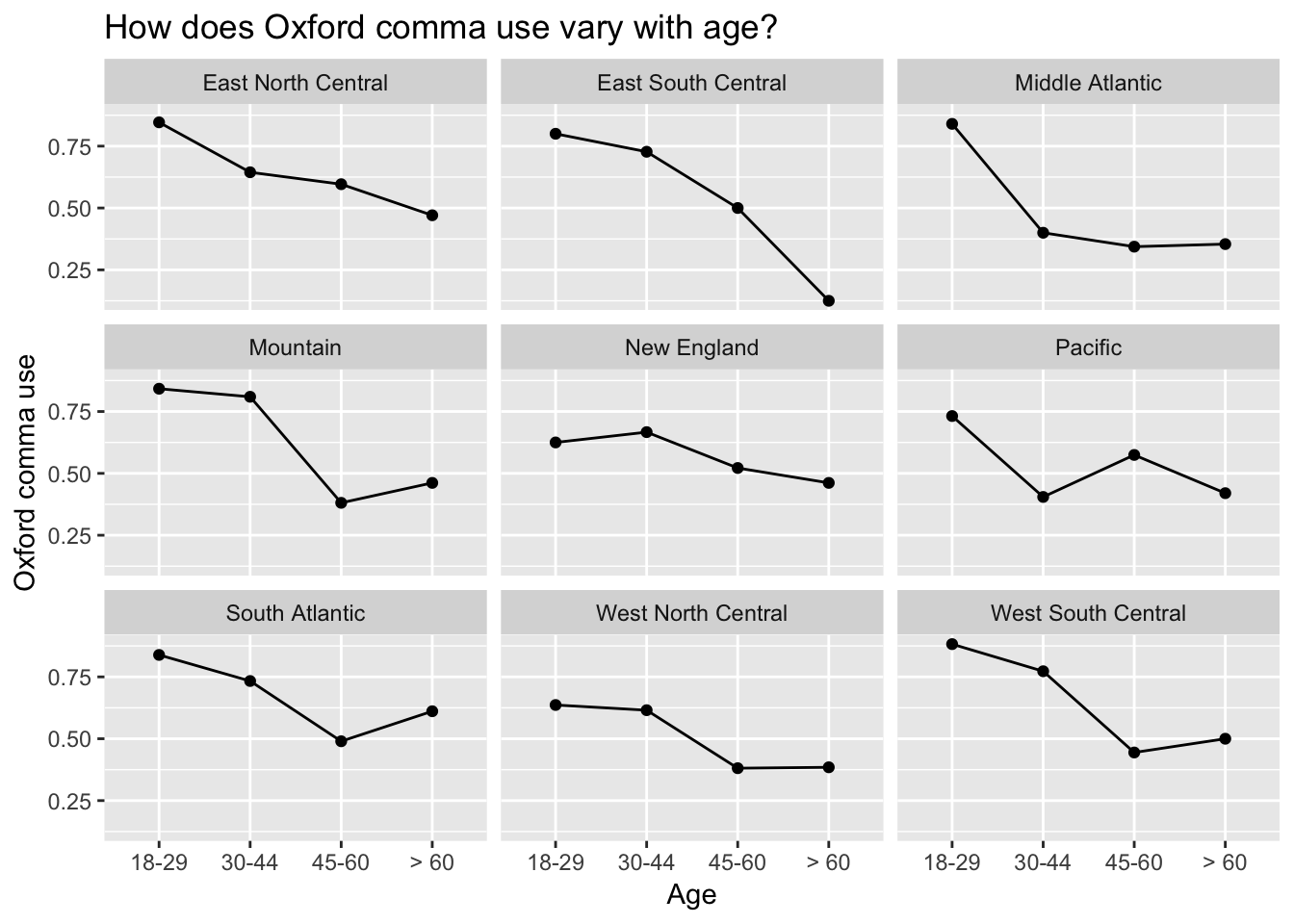Interestingly, in some places Oxford comma is much higher among younger respondents than older respondents, while in other places it’s use is relatively constant across all age groups.

What would we do if we wanted the different locations to appear in different places in this grid?

**Note that you can also facet by more than one variable (e.g. x and y) by using facet_grid(x ~ y).

# Beautifying your graph with THEMES!

This website has a full list of the different themes you can use in ggplot: https://ggplot2.tidyverse.org/reference/theme.html . This website has a list of colors you can use: http://sape.inf.usi.ch/quick-reference/ggplot2/colour. It can be helpful to refer to both of these while you’re working on editing a plot.

In this section, we’ll begin to dive into `ggplot()` themes, which allow you to control the appearance of your plot. Using themes, we can change the position, color, spacing, font, size, etc. of all aspects of the plot.

We’re going to work with the plot we saved above (called `plot.agelocation`).

``````# Start by calling the original plot:
plot.agelocation

# Next, change the size of the title font:
# Note that, for any change to text, we need to specify "element_text"
plot.agelocation + theme(title = element_text(size=14))

# We can also change multiple things at once:

plot.agelocation + theme(# Change all text to Times New Roman, size 12
text = element_text(size=12, family = "Times"),
# Change the background color of the panels:
panel.background = element_rect(fill="white"),
# Add light gray horizontal gridlines:
panel.grid.major.y = element_line(color="lightgrey"),
# Change the facet lables to a light blue background:
strip.background = element_rect(fill = "lightblue"))``````

# The magic of ggthemes()

Have you ever wanted to re-create the appearance of plots you’ve seen in different publications? The package `ggthemes()` used `ggplot()` themes to replicate the appearance of plots from a number of recognizable publications and programs. You can install the package using the code `install.packages("ggthemes")`. Then load it as follows:

``library(ggthemes)``

Do you recognize the plot formats below? You can also play around with other options by typing “theme_” into R – this will show you the other options built into `ggthemes().`

``plot.agelocation + theme_economist()``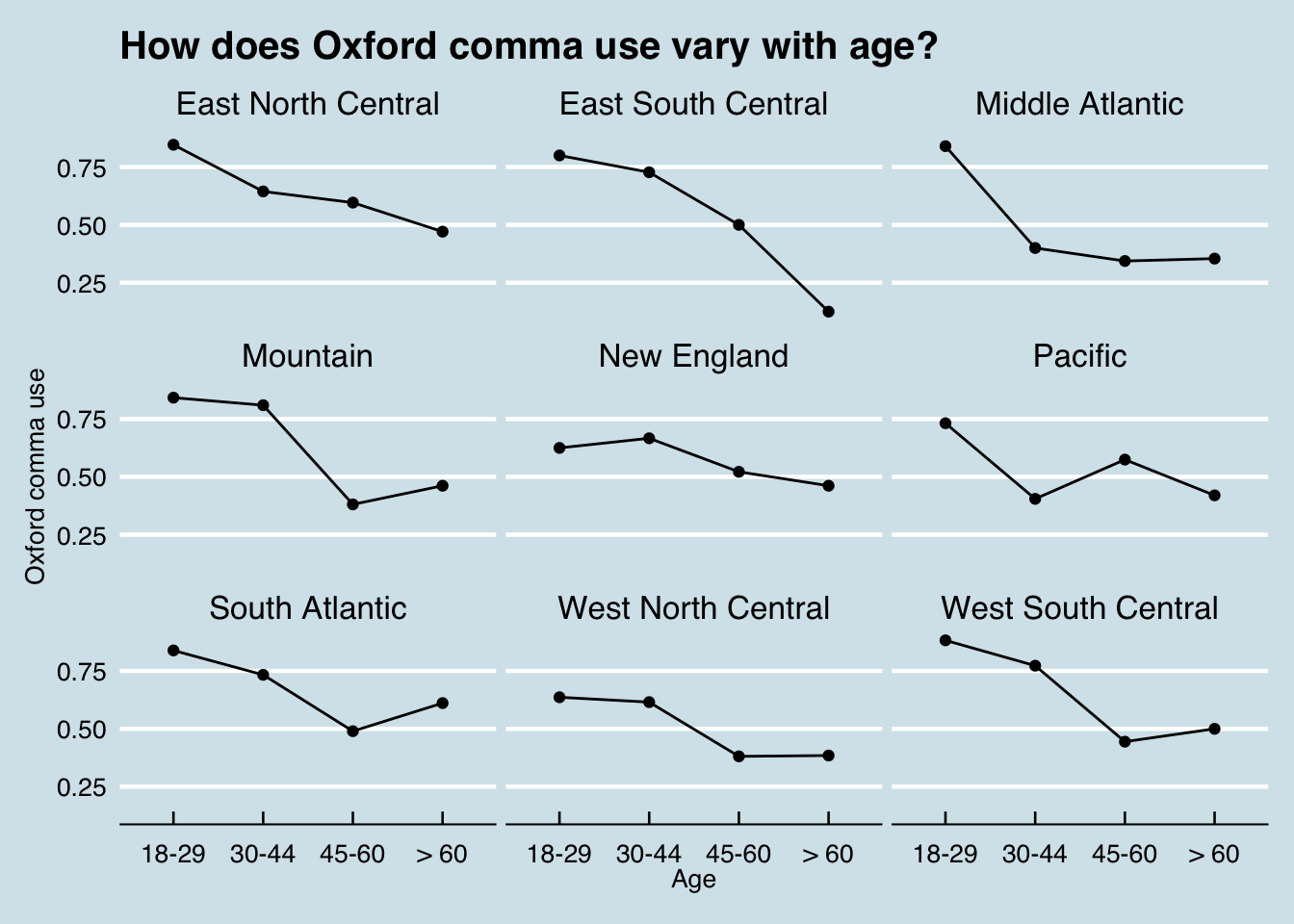``plot.agelocation + theme_stata()``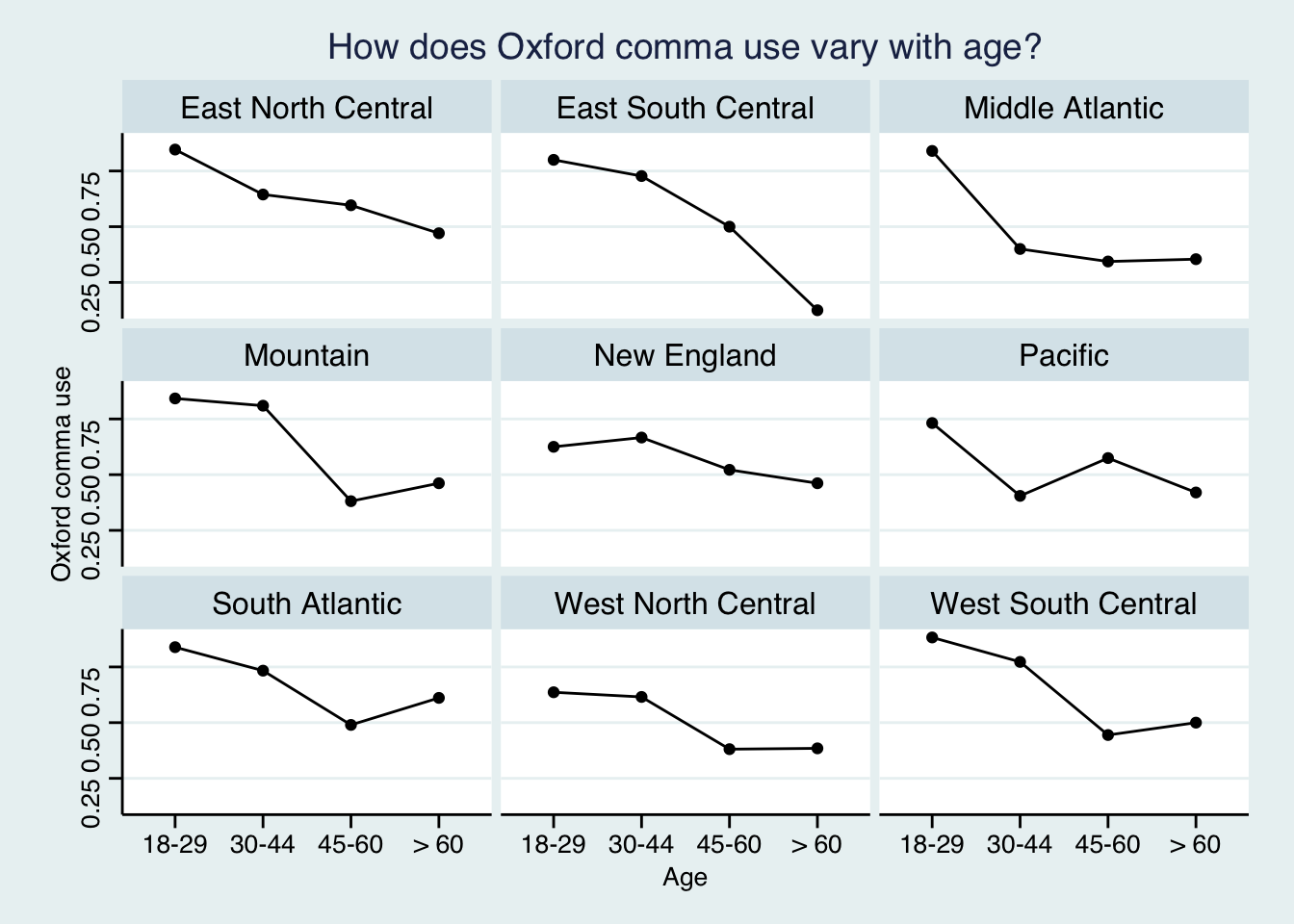``plot.agelocation + theme_wsj() + theme(title=element_text(size=16))``